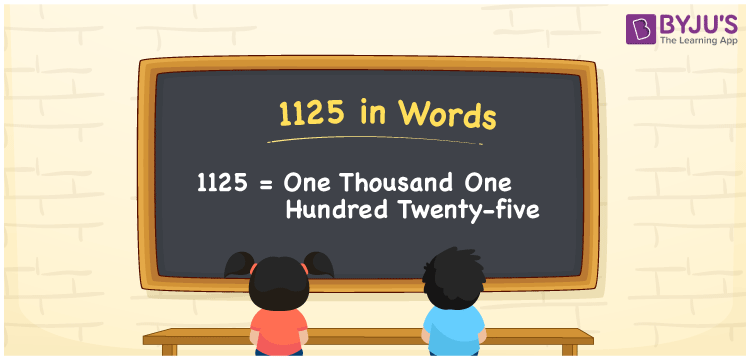# 1125 in Words

1125 in words is written as “One Thousand One Hundred Twenty-Five”. In Maths, 1125 is a cardinal number that expresses a quantity. To write a cheque for Rs.1125, we can write in words as “Rupees One Thousand One Hundred Twenty-Five only”. Learn more about Numbers In Words and writing the number names in English at BYJU’S.

 1125 in Words One Thousand One Hundred Twenty-Five One Thousand One Hundred Twenty-Five in Numerals 1125

## 1125 in English Words

To make it easy for children to learn the names of the numbers we have represented the chart below. It shows the name of 1125 in English words.## How to Write 1125 in Words?

The number 1125 in words can be written using a place value system, where we can identify the position of each digit of the original number. Since 1125 is a four-digit number, thus,

 Thousands Hundreds Tens Ones 1 1 2 5

From the above table,

1 → Thousands

1 → Hundreds

2 → Tens

5 → Ones

Hence, when we read the number from right to left, it is given by One Thousand One Hundred Twenty-Five.

### Expanded Form of 1125

We can write the expanded form as:

1 x Thousand + 1 × Hundred + 2 × Ten + 5 × One

= 1 x 1000 + 1 × 100 + 2 × 10 + 5 × 1

= 1000 + 100 + 20 + 5

= One Thousand One Hundred Twenty-Five

1125 is a whole number that is succeeded by 1124 and preceded by 1126. Learn more about the number 1125 below:

• 1125 in Words – One Thousand One Hundred Twenty-Five
• Is 1125 an odd number? – Yes
• Is 1125 an even number? – No
• Is 1125 a perfect square number? – No
• Is 1125 a perfect cube number? – No
• Is 1125 a prime number? – No
• Is 1125 a composite number? – Yes

## Frequently Asked Questions on 1125 in words

Q1

### What is 1125 in words?

1125 in words is given by One Thousand One Hundred Twenty-Five.
Q2

### What is the rule to write 1125 in words?

To write 1125 in words, we should use the place value rule, where the position of each digit helps in determining the name of the number.
Q3

### What is the value of 1125 + 100 in words?

1125 + 100 = 1225, i.e., One Thousand Two Hundred Twenty-Five in words.
Test your Knowledge on 1125 in Words